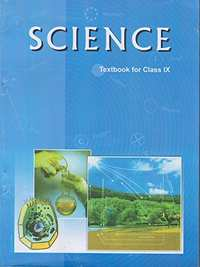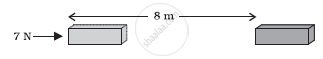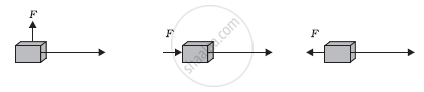Share

# NCERT solutions for Class 9 Science Textbook chapter 11 - Work and Energy [Latest edition]

Course
Textbook page

#### Chapters## Chapter 11: Work and Energy

#### NCERT solutions for Class 9 Science Textbook Chapter 11 Work and Energy [Page 148]

Q 1 | Page 148

A force of 7 N acts on an object. The displacement is, say 8 m, in the direction of the force (see the given figure). Let us take it that the force acts on the object through the displacement. What is the work done in this case?#### NCERT solutions for Class 9 Science Textbook Chapter 11 Work and Energy [Page 149]

Q 1 | Page 149

When do we say that work is done?

Q 2 | Page 149

Write an expression for the work done when a force is acting on an object in the direction of its displacement.

Q 3 | Page 149

Define 1 J of work.

Q 4 | Page 149

A pair of bullocks exerts a force of 140 N on a plough. The field being ploughed is 15 m long. How much work is done in ploughing the length of the field?

#### NCERT solutions for Class 9 Science Textbook Chapter 11 Work and Energy [Page 152]

Q 1 | Page 152

What is the kinetic energy of an object?

Q 2 | Page 152

Write an expression for the kinetic energy of an object.

Q 3 | Page 152

The kinetic energy of an object of mass, m moving with a velocity of 5 m s−1 is 25 J. What will be its kinetic energy when its velocity is doubled? What will be its kinetic energy when its velocity is increased three times?

#### NCERT solutions for Class 9 Science Textbook Chapter 11 Work and Energy [Page 156]

Q 1 | Page 156

What is power?

Q 2 | Page 156

Define 1 watt of power.

Q 3 | Page 156

A lamp consumes 1000 J of electrical energy in 10 s. What is its power?

Q 4 | Page 156

Define average power.

#### NCERT solutions for Class 9 Science Textbook Chapter 11 Work and Energy [Pages 158 - 159]

Q 1 | Page 158

Look at the activities listed below. Reason out whether or not work is done in the light of your understanding of the term ‘work’.

• Suma is swimming in a pond.
• A donkey is carrying a load on its back.
• A wind mill is lifting water from a well.
• A green plant is carrying out photosynthesis.
• An engine is pulling a train.
• Food grains are getting dried in the sun.
• A sailboat is moving due to wind energy.
Q 2 | Page 158

An object thrown at a certain angle to the ground moves in a curved path and falls back to the ground. The initial and the final points of the path of the object lie on the same horizontal line. What is the work done by the force of gravity on the object?

Q 3 | Page 158

A battery lights a bulb. Describe the energy changes involved in the process.

Q 4 | Page 158

Certain force acting on a 20 kg mass changes its velocity from 5 m s−1 to 2 m s−1. Calculate the work done by the force.

Q 5 | Page 158

A mass of 10 kg is at a point A on a table. It is moved to a point B. If the line joining A and B is horizontal, what is the work done on the object by the gravitational force? Explain your answer.

Q 6 | Page 158

The potential energy of a freely falling object decreases progressively. Does this violate the law of conservation of energy? Why?

Q 7 | Page 158

What are the various energy transformations that occur when you are riding a bicycle?

Q 8 | Page 158

Does the transfer of energy take place when you push a huge rock with all your might and fail to move it? Where is the energy you spend going?

Q 9 | Page 158

A certain household has consumed 250 units of energy during a month. How much energy is this in joules?

Q 10 | Page 158

An object of mass 40 kg is raised to a height of 5 m above the ground. What is its potential energy? If the object is allowed to fall, find its kinetic energy when it is half-way down.

Q 11 | Page 158

What is the work done by the force of gravity on a satellite moving round the earth? Justify your answer.

Q 12 | Page 158

Can there be displacement of an object in the absence of any force acting on it? Think. Discuss this question with your friends and teacher.

Q 13 | Page 159

A person holds a bundle of hay over his head for 30 minutes and gets tired. Has he done some work or not? Justify your answer.

Q 14 | Page 159

An electric heater is rated 1500 W. How much energy does it use in 10 hours?

Q 15 | Page 159

Illustrate the law of conservation of energy by discussing the energy changes which occur when we draw a pendulum bob to one side and allow it to oscillate. Why does the bob eventually come to rest? What happens to its energy eventually? Is it a violation of the law of conservation of energy?

Q 16 | Page 159

An object of mass, m is moving with a constant velocity, v. How much work should be done on the object in order to bring the object to rest?

Q 17 | Page 159

Calculate the work required to be done to stop a car of 1500 kg moving at a velocity of 60 km/h?

Q 18 | Page 159

In each of the following a force, F is acting on an object of mass, m. The direction of displacement is from west to east shown by the longer arrow. Observe the diagrams carefully and state whether the work done by the force is negative, positive or zero.Q 19 | Page 159

Soni says that the acceleration in an object could be zero even when several forces are acting on it. Do you agree with her? Why?

Q 20 | Page 159

Find the energy in kW h consumed in 10 hours by four devices of power 500 W each.

Q 21 | Page 159

A freely falling object eventually stops on reaching the ground. What happens to its kinetic energy?

## Chapter 11: Work and Energy## NCERT solutions for Class 9 Science Textbook chapter 11 - Work and Energy

NCERT solutions for Class 9 Science Textbook chapter 11 (Work and Energy) include all questions with solution and detail explanation. This will clear students doubts about any question and improve application skills while preparing for board exams. The detailed, step-by-step solutions will help you understand the concepts better and clear your confusions, if any. Shaalaa.com has the CBSE Class 9 Science Textbook solutions in a manner that help students grasp basic concepts better and faster.

Further, we at Shaalaa.com provide such solutions so that students can prepare for written exams. NCERT textbook solutions can be a core help for self-study and acts as a perfect self-help guidance for students.

Concepts covered in Class 9 Science Textbook chapter 11 Work and Energy are Work and Energy (Introduction ), Scientific Conception of Work, Work Done by a Constant Force, Work Done by a Energy, Work Done by a Power, Different Forms of Energy, Kinetic Energy, Potential Energy, Potential Energy of an Object at a Height, Are Various Energy Forms Interconvertible?, Law of Conservation of Energy, Rate of Doing Work, Commercial Unit of Energy, Work and Energy (Numericals).

Using NCERT Class 9 solutions Work and Energy exercise by students are an easy way to prepare for the exams, as they involve solutions arranged chapter-wise also page wise. The questions involved in NCERT Solutions are important questions that can be asked in the final exam. Maximum students of CBSE Class 9 prefer NCERT Textbook Solutions to score more in exam.

Get the free view of chapter 11 Work and Energy Class 9 extra questions for Class 9 Science Textbook and can use Shaalaa.com to keep it handy for your exam preparation

S